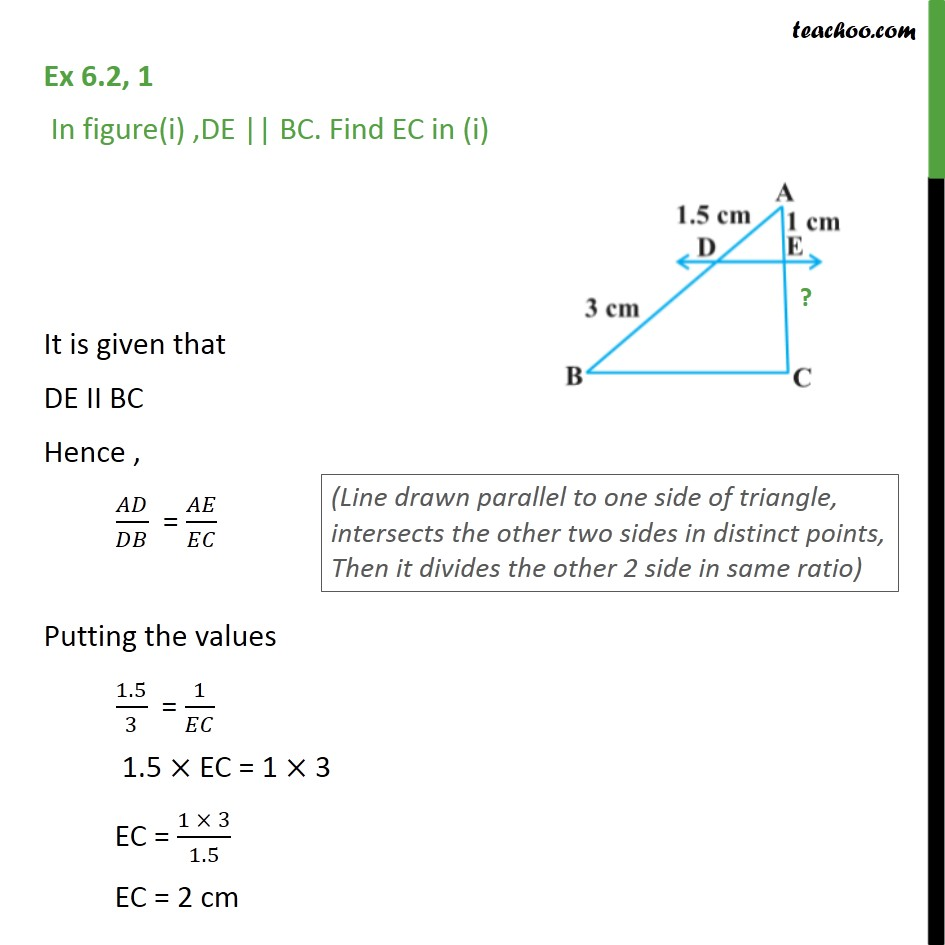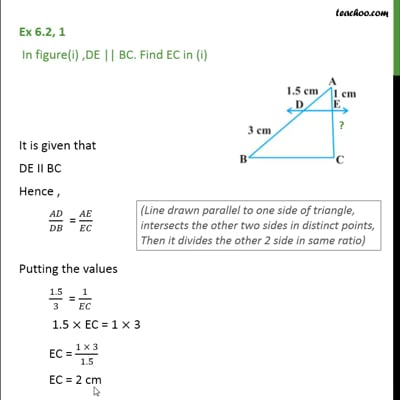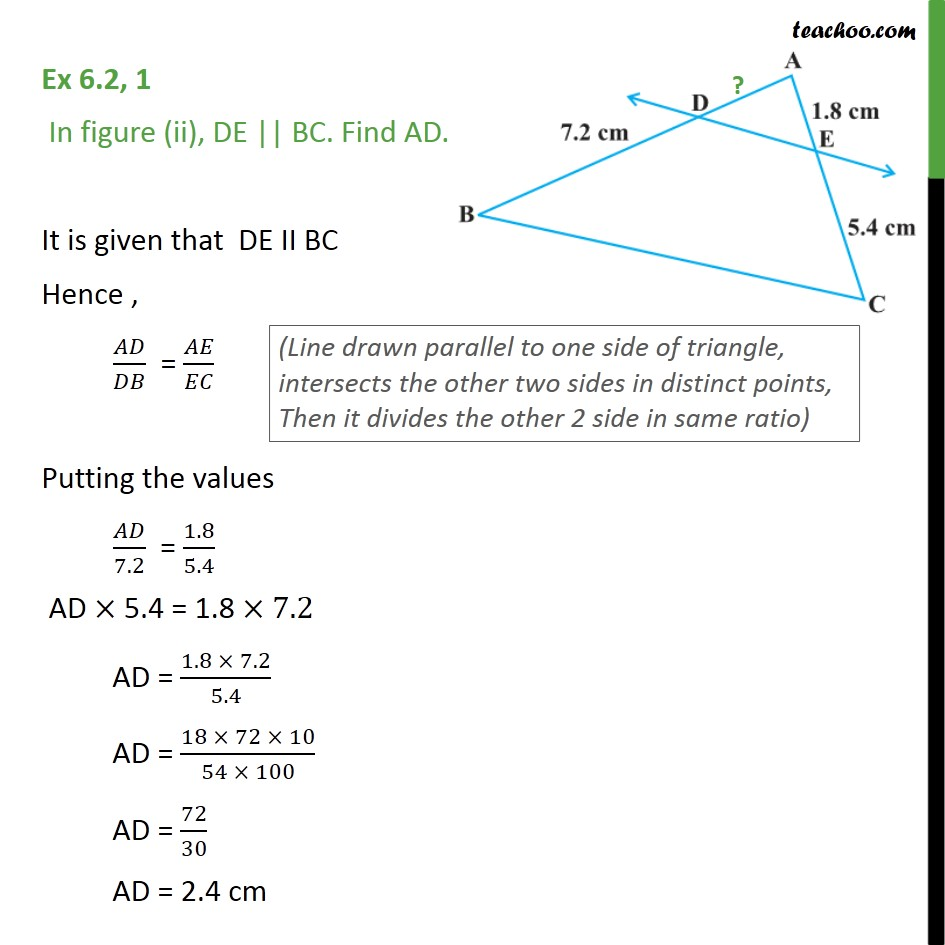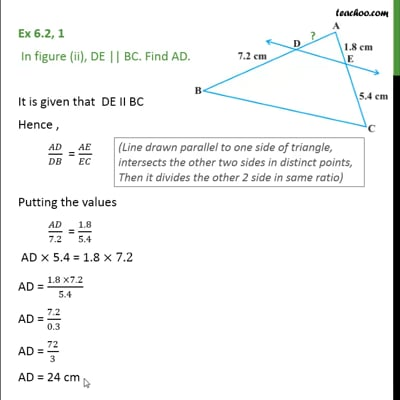Ex 6.2

Chapter 6 Class 10 Triangles (Term 1)
Serial order wiseThis video is only available for Teachoo black usersThis video is only available for Teachoo black users

### Transcript

Ex 6.2, 1 In figure(i) ,DE || BC. Find EC in (i) It is given that DE II BC Hence , / = / Putting the values 1.5/3 = 1/ 1.5 EC = 1 3 EC = (1 3)/1.5 EC = 2 cm Ex 6.2, 1 In figure (ii), DE || BC. Find AD. It is given that DE II BC Hence , 𝐴𝐷/𝐷𝐵 = 𝐴𝐸/𝐸𝐶 Putting the values 𝐴𝐷/7.2 = 1.8/5.4 AD × 5.4 = 1.8 ×7.2 AD = (1.8 × 7.2)/5.4 AD = (18 × 72 × 10)/(54 × 100) AD = 72/30 AD = 2.4 cm